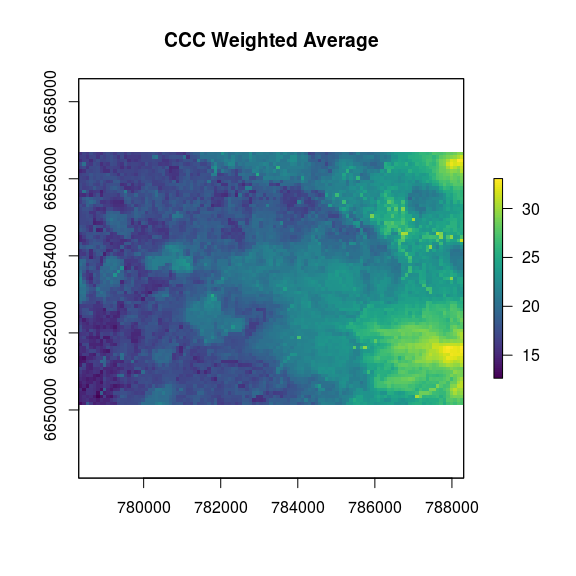# Dissever Tutorial

#### 2018-04-20

library(dissever)

library(raster)
library(viridis)
library(dplyr)
library(magrittr)

Here is the coarse model to dissever:

plot(edgeroi$carbon, col = viridis(100))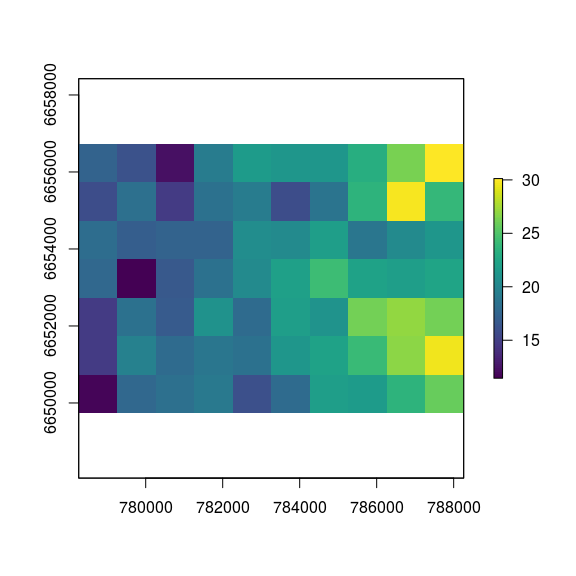and here are the covariates that will be used for the disseveration: plot(edgeroi$predictors, col = viridis(100))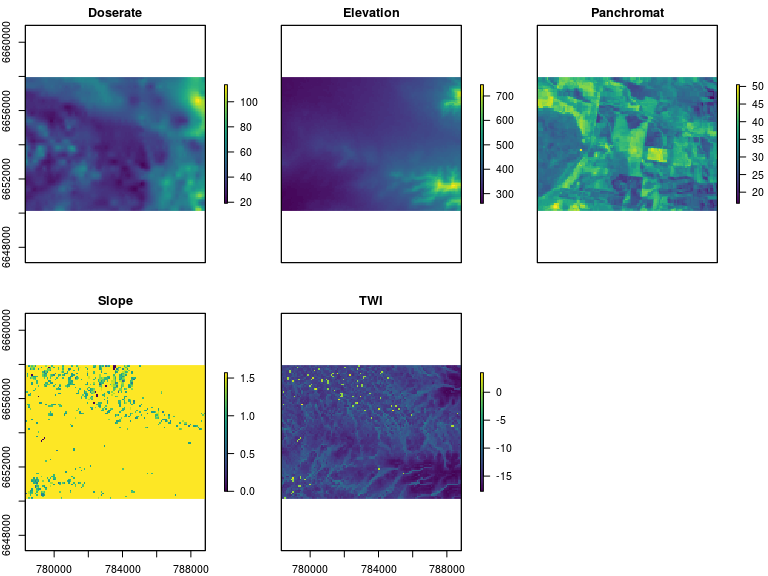## Example

In this section, we will dissever the sample dataset with the available environmental covariates, using 4 different regression methods:

• Random Forest ("rf")
• Cubist ("cubist")
• MARS ("earth")
• Linear Model ("lm")

It is simple to switch between regression methods using the method option of dissever.

But first let’s setup some parameters:

min_iter <- 5 # Minimum number of iterations
max_iter <- 10 # Maximum number of iterations
p_train <- 0.025 # Subsampling of the initial data

We can then launch the 4 different disseveration procedures:

# Random Forest
res_rf <- dissever(
coarse = edgeroi$carbon, # stack of fine resolution covariates fine = edgeroi$predictors, # coarse resolution raster
method = "rf", # regression method used for disseveration
p = p_train, # proportion of pixels sampled for training regression model
min_iter = min_iter, # minimum iterations
max_iter = max_iter # maximum iterations
)

# Cubist
res_cubist <- dissever(
coarse = edgeroi$carbon, fine = edgeroi$predictors,
method = "cubist",
p = p_train,
min_iter = min_iter,
max_iter = max_iter
)

# GAM
res_gam <- dissever(
coarse = edgeroi$carbon, fine = edgeroi$predictors,
method = "gamSpline",
p = p_train,
min_iter = min_iter,
max_iter = max_iter
)

# Linear model
res_lm <- dissever(
coarse = edgeroi$carbon, fine = edgeroi$predictors,
method = "lm",
p = p_train,
min_iter = min_iter,
max_iter = max_iter
)

# Analysing the results

The object returned by dissever is an object of class dissever. It’s basically a list with 3 elements: * fit: a train object storing the regression model used in the final disseveration * map: a RasterLayer object storing the dissevered map * perf: a data.frame object storing the evolution of the RMSE (and confidence intervals) with the disseveration iterations.

The dissever result has a plot function that can plot either the map or the performance results, depending on the type option.

## Maps

Below are the dissevered maps for all 4 regression methods:

# Plotting maps
par(mfrow = c(2, 2))
plot(res_rf, type = 'map', main = "Random Forest")
plot(res_cubist, type = 'map', main = "Cubist")
plot(res_gam, type = 'map', main = "GAM")
plot(res_lm, type = 'map', main = "Linear Model")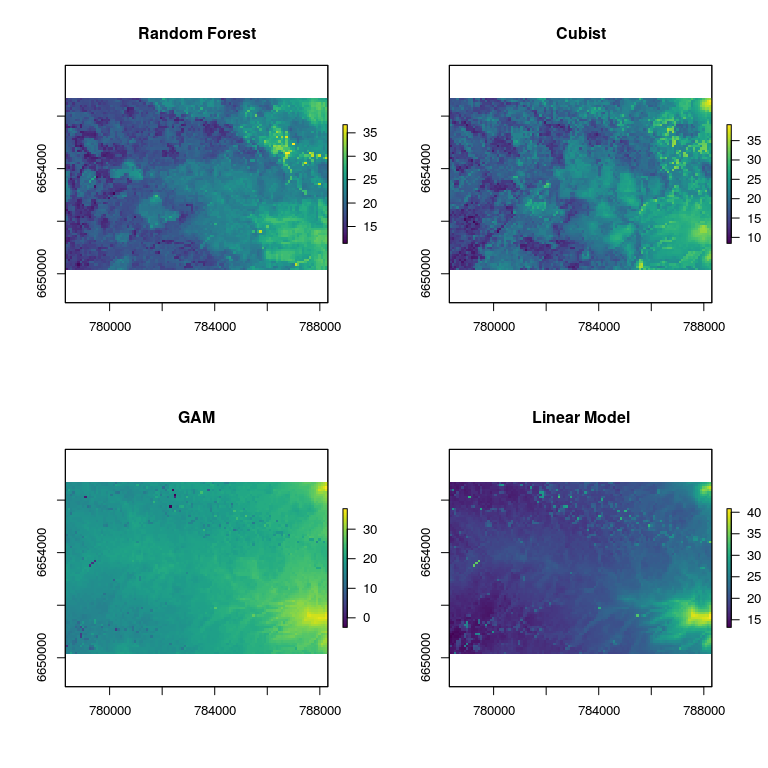## Convergence

We can also analyse and plot the optimisation of the dissevering model:

# Plot performance
par(mfrow = c(2, 2))
plot(res_rf, type = 'perf', main = "Random Forest")
plot(res_cubist, type = 'perf', main = "Cubist")
plot(res_gam, type = 'perf', main = "GAM")
plot(res_lm, type = 'perf', main = "Linear Model")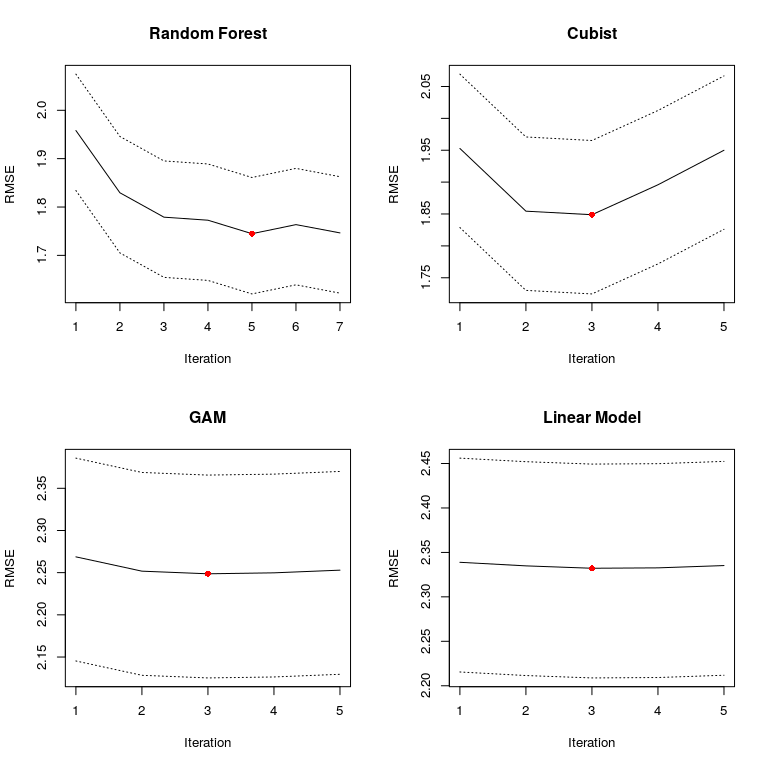## Performance of the regression

The tools provided by the caret package can also be leveraged, e.g. to plot the observed vs. predicted values:

# Plot preds vs obs
preds <- extractPrediction(list(res_rf$fit, res_cubist$fit, res_gam$fit, res_lm$fit))
plotObsVsPred(preds)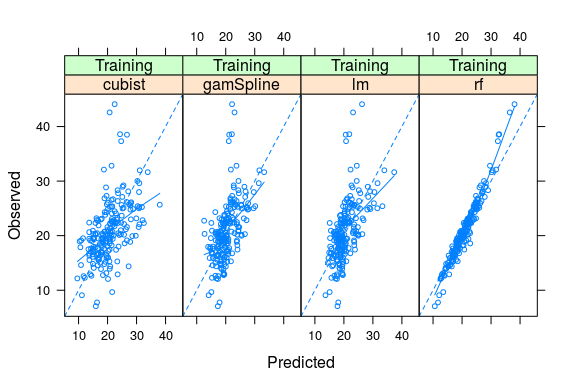The models can be compared using the preds object that we just derived using the extractPrediction function. In this case I’m computing the R2, the RMSE and the CCC for each model:

# Compare models
perf <- preds %>%
group_by(model, dataType) %>%
summarise(
rsq = cor(obs, pred)^2,
rmse = sqrt(mean((pred - obs)^2))
)
perf
## # A tibble: 4 x 4
## # Groups:   model [?]
##   model     dataType   rsq  rmse
##   <fct>     <fct>    <dbl> <dbl>
## 1 cubist    Training 0.260  5.35
## 2 gamSpline Training 0.312  4.66
## 3 lm        Training 0.349  4.52
## 4 rf        Training 0.951  1.53

# Ensemble approach

We can either take the best option (in this case Random Forest – but the results probably show that we should add some kind of validation process), or use a simple ensemble-type approach. For the latter, I am computing weights for each of the maps based on the CCC of the 4 different regression models:

# We can weight results with Rsquared
w <- perf$rsq / sum(perf$rsq)

# Make stack of weighted predictions and compute sum
l_maps <- list(res_cubist$map, res_gam$map, res_lm$map, res_rf$map)
ens <- lapply(1:4, function(x) l_maps[[x]] * w[x]) %>%
stack %>%
sum

Ensemble modelling seem to work better when the models are not too correlated – i.e. when they capture different facets of the data:

s_res <- stack(l_maps)
names(s_res) <- c('Cubist', 'GAM', 'Linear Model', 'Random Forest')
s_res %>%
as.data.frame %>%
na.exclude %>%
cor
##                  Cubist       GAM Linear.Model Random.Forest
## Cubist        1.0000000 0.7725831    0.7027710     0.7421504
## GAM           0.7725831 1.0000000    0.8880034     0.7831751
## Linear.Model  0.7027710 0.8880034    1.0000000     0.8094299
## Random.Forest 0.7421504 0.7831751    0.8094299     1.0000000

Here is the resulting map:

# Plot result
plot(ens, col = viridis(100), main = "CCC Weighted Average")